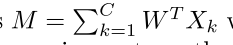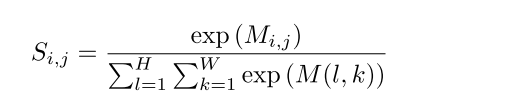# How to code attention map equation in Pytorch

I am trying to code a model which works with a feature map to end up with an attention map. I think I got some progress in coding it but I am stuck in getting the final attention (attn) calculation.

Some definitions:

``````Xk is the kth channel of the input feature maps X after going through a ResNet18. X shape is C x H x W
W is the weights of dilated convolution blocks after X is sent through 2 of them.
M is output of compressing features by computing the sum of all channels
S is generated by applying a spatial softmax layer that performs the softmax operation over all feature points in the aggregated map M
XT is the original convolutional feature maps with the shape of c × hw
``````

I am stuck trying to figure out how to code the following equation :The rest of the equations are:The code I have upto now

``````def forward(self, x):
# x.shape - torch.Size([1, 3, 500, 500])
X = self.resnet18(x) # torch.Size([1, 512, 32, 32])
W = self.dialation_network(X) # torch.Size([1, 512, 32, 32])
M = torch.einsum('bchw,bchw->bhw', W, X) # torch.Size([1, 32, 32])
S = F.softmax(M.reshape(1, -1), dim=1) #torch.Size([1, 1024])
XT = Xk.reshape(1, 512, 32*32) # torch.Size([1, 512, 1024])
attn = # TODO

return out
``````

I would like to know whether,

1. What I have done upto now is correct
2. How to get the final equation in pytorch

Any help is appreciated!

TIA!